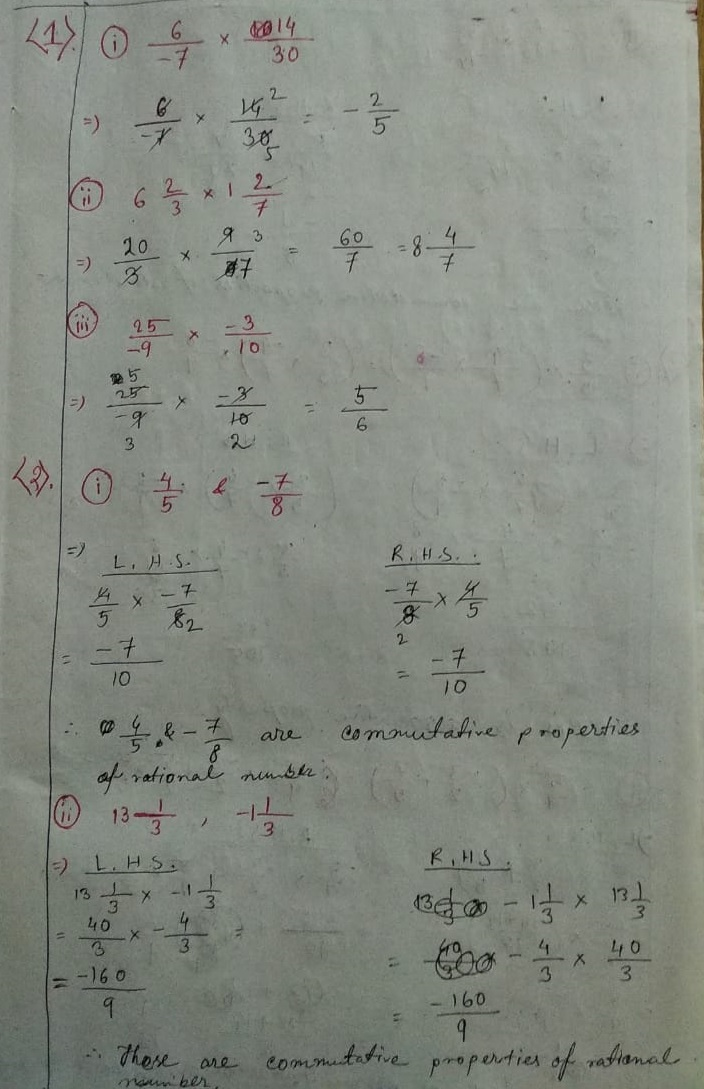# ML Aggarwal CBSE Solutions Class 8 Math First Chapter Rational Numbers Exercise 1.3

### ML Aggarwal CBSE Solutions Class 8 Math 1st Chapter Rational Numbers Exercise 1.3(4) (i) 12

=) The multiplicative inverse of 12 is 1/12

(ii) 2/3

=) The multiplicative inverse of 2/3 is 3/2

(iii) -4/7

=) The multiplicative inverse of -4/7 is 7/-4

(iv) -3/8 x (-7/13)

=) -3/8 x -7/13

= 21/104

The multiplicative inverse of 21/104 is 104/21(7) (i) Zero has no reciprocal

(ii) 1, (-1)

(iii) x2

(iv) 1

(v) a negative rational number(9) The additive inverse of 9 = – 9

The multiplicative inverse of 9 = 1/9

∴ Sum of additive inverse & multiplicative inverse

= -9 + 1/9

= -81+1/9

= -82/9

= -8~1/9

(10) The additive inverse of -3/7 = -(–3/7)

The multiplicative inverse of -3/7 = -7/3

∴ Sum of both = 3/7 x (-7/3)

= -1

Updated: February 15, 2021 — 5:39 pm